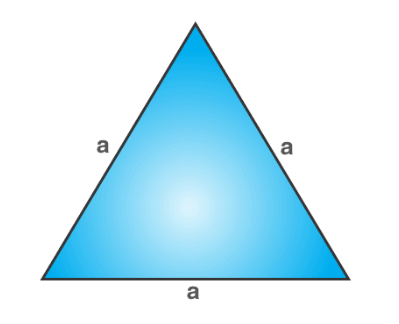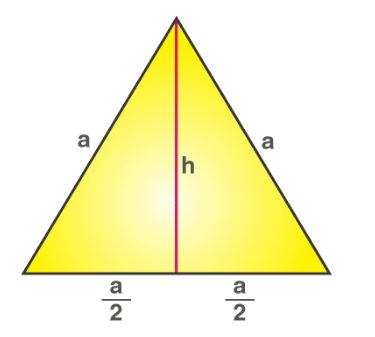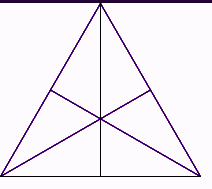In geometry, an equilateral triangle is a triangle that has all its sides equal in length. Since the three sides are equal therefore the three angles, opposite to the equal sides, are equal in measure. Therefore, it is also called an equiangular triangle, where each angle measure 60 degrees. Just like other types of triangles, an equilateral triangle also has its area, perimeter and height formula.  Let us learn more in this article.

## What is an Equilateral Triangle?

As we have already discussed in the introduction, an equilateral triangle is a triangle that has all its sides equal in length. Also, the three angles of the equilateral triangle are congruent and equal to 60 degrees. The sum of all three angles of an equilateral triangle is equal to 180 degrees.  60° + 60° + 60° = 180°. Thus, it obeys the angle sum property of triangle## Shape of Equilateral Triangle

The shape of an equilateral triangle is regular. The word ‘Equilateral’ is formed by the combination of two words, i.e., “Equi” meaning equal and “Lateral” meaning sides. An equilateral triangle is also called a regular polygon or regular triangle since all its sides are equal.

Suppose, ABC is an equilateral triangle, then, as per the definition;

AB = BC = AC, where AB, BC and AC are the sides of the equilateral triangle.

And

∠A = ∠B = ∠C = 60°

Based on sides there are other two types of triangles:

## Properties of Equilateral Triangle

• All three sides are equal.
• All three angles are congruent and are equal to 60 degrees.
• It is a regular polygon with three sides.
• The perpendicular drawn from vertex of the equilateral triangle to the opposite side bisects it into equal halves. Also, the angle of the vertex from where the perpendicular is drawn is divided into two equal angles, i.e. 30 degrees each.
• The ortho-centre and centroid are at the same point.
• In an equilateral triangle, median, angle bisector, and altitude for all sides are all the same.
• The area of an equilateral triangle is √3a2/ 4
• The perimeter of an equilateral triangle is 3a.

## Comparison: Scalene, Isosceles and Equilateral Triangles

 Equilateral triangle Isosceles triangle Scalene triangle All three sides are equal Any two sides are equal All three sides are unequal All the three interior angles are equal to 60 degrees Angles opposite to equal sides are equal All the angles are unequal in measure

## Equilateral Triangle Theorem

If ABC is an equilateral triangle and P is a point on the arc BC of the circumcircle of the triangle ABC, then;

PA = PB + PC

Proof: For a cyclic quadrilateral ABPC, we have;

PA⋅BC=PB⋅AC+PC⋅AB

Since we know, for an equilateral triangle ABC,

AB = BC = AC

Therefore,

PA.AB = PB.AB+PC.AB

Taking AB as a common;

PA.AB=AB(PB+PC)

PA = PB + PC

Hence, proved.

## Equilateral Triangle Formulas

We have already understood an equilateral triangle has all three sides equal in length and all three angles equal in measure. Now based on these properties the formulas for equilateral triangles are defined. The most common formulas that we consider for a triangle are:

• Area of equilateral triangle
• Perimeter of equilateral triangle
• Height of equilateral triangle

In the next section, we will be discussing all these formulas.

## Area of Equilateral Triangle

The area of an equilateral triangle is the region occupied by it in a two-dimensional plane. The formula for the area of an equiangular triangle is given by:

A = √3a2/4

Let us derive the formula here:If we see the above figure, the area of a triangle is given by;

Area = ½ x base x height

Here Base = a and height = h

Therefore,

Area = ½ x a x h ………(1)

Now, from the above figure, the altitude h bisects the base into equal halves, such as a/2 and a/2. It also forms two equivalent right-angled triangles.

So, for a right triangle, using Pythagoras theorem, we can write:

a2 = h2 + (a/2)2

or

h2 = (a)2 – (a/2)2

= 3a2/4

h = √3a/2

By putting this value in equation 1, we get;

Area = ½ x a x √3a/2

A = √3a2/4

Hence, the area of the equilateral triangle equals to √3a2/4.

## Perimeter of Equilateral Triangle

In geometry, the perimeter of any polygon is equal to the length of its sides. In the case of the equilateral triangle, the perimeter will be the sum of all three sides.

Suppose, ABC is an equilateral triangle, then the perimeter of ∆ABC is;

Perimeter = AB + BC + AC

P = a + a + a

P = 3a

Where a is the length of sides of the triangle.

## Height of Equilateral Triangle

The height of an equilateral triangle can be determined using the Pythagoras theorem. It is also called altitude of an equilateral triangle. As we know, an equilateral triangle has all equal sides. Now, if we drop an altitude from the apex of the triangle to the base, it divides the triangle into two equal right triangles.

Thus, from the above figure, we can find the height (h) of the equilateral triangle, as:

h = √3a/2

Where a is the side of the triangle.

Thus, to summarise the formulas related to equilateral triangle are:

 Area √3a2/4 Perimeter 3a Height √3a/2

## Centroid of Equilateral Triangle

The centroid of the equilateral triangle lies at the center of the triangle. Since all its sides are equal in length, hence it is easy to find the centroid for it.

To find the centroid, we need to draw perpendiculars from each vertex of the triangle to the opposite sides. These perpendiculars are all equal in length and intersect each other at a single point, which is known as centroid. See the figure below:Note: The centroid of a regular triangle is at equidistant from all the sides and vertices.

## Circumcenter

The circumcenter of equilateral triangle is the point of intersection perpendicular bisectors of the sides. Here, the circumcircle passes through all the three vertices of the triangle.

If any of the incenter, orthocenter or centroid coincide with the circumcenter of a triangle, then it is called an equilateral triangle.

 Facts of Equilateral Triangle: Number of Sides = 3 Number of angles = 3 Each interior angle = 60 Each exterior angle = 120 Perimeter = 3 times of side-length Area = √3/ 4 x (side)2 Height = √3 (side)/2

## Solved Examples on Equilateral Triangle

Q.1: Find the area of the equilateral triangle ABC, where AB=AC=BC = 4cm.

Solution:

By the formula, we know;

Area = √3a2/4

Given a = 4cm

Hence, by putting the value we get;

Area = √3(4)2/4

A = 4√3

Q.2: Find the altitude of an equilateral triangle whose sides are equal to 10cm.

Solution:

By the formula, we know,

Height of an equilateral triangle = √3a/2

Since, a = 10cm

Hence,

h = √3 x (10/2)

h = 5√3

### Video Lesson on Types of TrianglesFor more related articles, register with BYJU’S.

## Frequently Asked Questions on Equilateral Triangle

### What is an equilateral triangle?

A triangle that has all its sides equal in dimension and each angle measure up to 60 degrees, is called an equilateral triangle.

### What is the area of equilateral triangle formula?

The formula to find area of equilateral triangle is given by:
A = (√3/4)a2, where a is the length of side of equilateral triangle.

### What is the perimeter of an equilateral triangle?

The perimeter of an equilateral triangle is the sum of all its three equal sides.
Perimeter = 3a, where a is the length of its sides.

### What is the height of equilateral triangle?

The formula to find the height of equilateral triangle is:
Height, h = (√3/2)a, where a is the side of the equilateral triangle.

### What type of polygon is an equilateral triangle?

An equilateral triangle is a regular polygon or a regular triangle.

### What is the different name of triangles based on sides?

Based on sides, there are three different kinds of triangles. Their names are:
Scalene triangle
Isosceles triangle
Equilateral triangle

### How many sides does an equilateral triangle has?

All triangles have 3 sides only.

### What is the perimeter of equilateral triangle with side equal to 10cm?

Perimeter = 3 x sides of equilateral triangle
Perimeter = 3 x 10 = 30 cms

Test your knowledge on Equilateral Triangle

#### 1 Comment

1. BHARTI BAIRAGI

good content ever very helpful to learn.